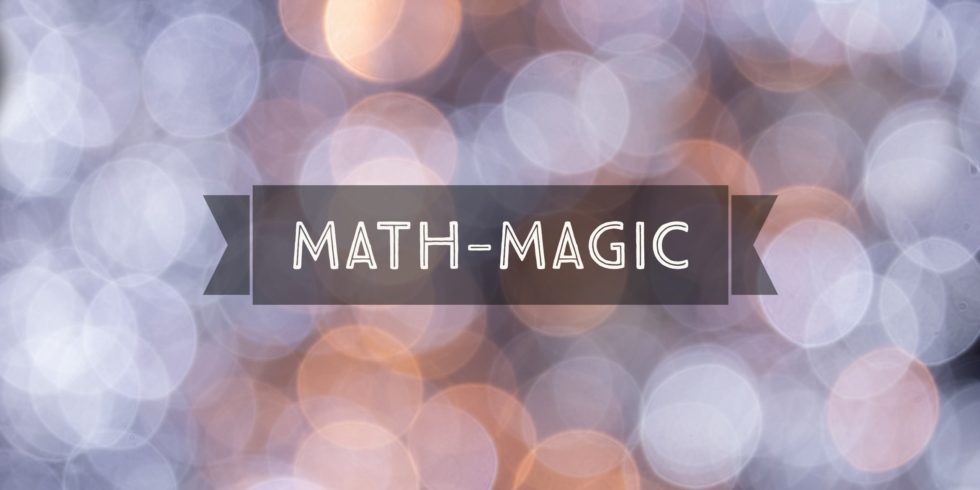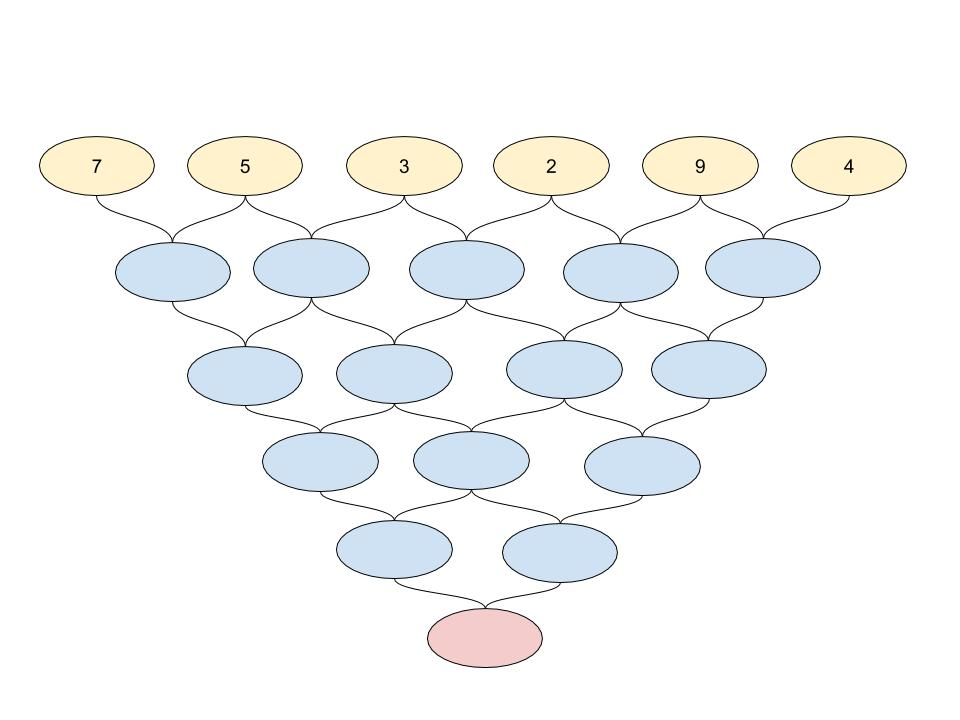# The Trick of a Mathematical Game. The One’s Digit of the Sum of Two Numbers.## Problem 521

Decipher the trick of the following mathematical magic.Add to solve later

## The Rule of the Game

Here is the game.

Pick six natural numbers ($1, 2, 3, \dots$) and place them in the yellow discs of the picture below.For example, let’s say I have chosen the numbers $7, 5, 3, 2, 9, 4$.The next step is adding the adjacent two numbers and put the one’s digit of the sum in the discs connecting the two.

For example, look at the top-left numbers $7$ and $5$.

The sum is $7+5=12$, and the one’s digit is $2$. So I put $2$ in the blue disc below the discs of $7$ and $5$.

Repeat this procedure: Summing two numbers next to each other and write down the one’s digit of the sum below.

The number on the last disc ( the red one) is your result.

With my choice $7, 5, 3, 2, 9, 4$, I getand the result is $1$.

This is the rule of the game.

## Lightning Speed Computation

Now, let play this game with you.

Suppose that you chose the numbers are $6, 3, 11, 5, 2, 1$.
Then you start the game. As soon as you write down the one’s digit of the first sum, I exclaim that the result is $2$!!
(We will come back to this after explaining the trick.)

How did I get the answer very quickly? Am I a computational generous that can calculate like a robot?

No.

There is a trick, a mathematical trick behind the game.

## A Secret Trick of the Mathematical Magic

Let me give the conclusion first.

Trick.
If the six numbers are $a, b, c, d, e, f$, then the result is given by the one’s digit of the number
$a+f+5(b+e).$

Furthermore,

• If $b+e$ is even, then the result is the one’s digit of $a+f$.
• If $b+e$ is odd, then the result is the one’s digit of $a+f+5$.

### Proof of the Trick

Let $a, b, c, d, e, f$ be the chosen six natural numbers.

Here is an important observation.
Even though we take the one’s digit after summing two numbers, the result will not change if we just sum numbers in each step except the last step (we take the one’s digit of the last entry).

So we have the tree diagramSo the result is the one’s digit of
$a+5b+10c+10d+5e+f.$

Note that the one’s digit of a number is the remainder when the number is divided by $10$.
(If you know modular arithmetic, then this means that we compute modulo $10$.)

Thus, $10c$ and $10d$ give no contribution to the remainder.

It follows that the result is the one’s digit of
$a+5b+5e+f=a+f+5(b+e).$

If $b+e$ is even, then $5(b+e)$ is a multiple of $10$.
Hence the one’s digit of $5(b+e)$ is zero.

Thus if $b+e$ is even, then the result is the one’s digit of $a+f$.

If $b+e$ is odd, then the one’s digit of $5(b+e)$ is always $5$.

Hence if $b+e$ is odd, then the result is the one’s digit of $a+f+5$.

This is the trick of the game.

An interesting observation is that the middle numbers $c, d$ do not appear in the formula.

## Example

Let’s go back the numbers $6, 3, 11, 5, 2, 1$.

In this case, $a=6, b=3, c=11, d=5, e=2, f=1$.

We compute
$a+f+5(b+e)=6+1+5(3+2)=7+25=32$ and the one’s digit of $32$ is $2$.

Thus the result is $2$ as I exclaimed before.

The faster way is to determine the parity of $b+e$ first.
Since $b+e=3+2=5$ is odd, the result is the one’s digit of $a+f+5=6+1+5$, and hence the result is $2$.

If you don’t know the formula then you would complete the tree diagram like this:and clearly it takes more time to get the result without the trick.

### Modulo $10$

Here is a tip to use the formula $a+f+5(b+e)$.

If the numbers are relatively small, then just compute it and find the one’s digit.

But remember that you can always do modulo $10$ computation anytime. (Considering the one’s digit or the remainder of division by $10$.)

For example, if $a=11, f=29, b=17, e=104$ are given, I first find the one’s digits of them and can assume that $a=1, f=9, b=7, e=4$.

Then $a+f=10$ and the one’s digit is $0$. So I can safely ignore the $a+f$ part in the formula.
Next, $b+e=11$ and the one’s digit is $1$.
(Or you could first determine the parity of $b+e$.)

Thus by the formula, the result is $5$.

## Do Practice and Have some Fun!

Practice how to use the formula several times and get used to it.Add to solve later